Vibroengineering PROCEDIA

Published: 19 October 2019

# Study on dynamic characteristic of closed-cell aluminum foam

Qiang Lei1
Junlong Ren2
Hongyi Ren3
Hongxiao Chao4
Wenbin Du5
1, 2, 3, 4, 5Northwest Institute of Mechanical and Electrical Engineering, 712099, Xianyang, P. R. China
Corresponding Author:
Qiang Lei
Views 59

#### Abstract

Closed-cell aluminum foam has been widely used in aerospace, rail transit and mechanical for its outstanding performance. But for a long time, the research on its vibration damping performance is only limited to the material damping test, there are relatively few studies on its dynamic characteristics. In this paper, we studied the relationship between dynamic characteristic and feature parameters. Modal assurance criterion and Finite element method were used to verify the accuracy of experimental model. It turned out that the average pore diameter of closed-cell aluminum foam conforms to Gaussian distribution. The modal analysis method can be used in the research of dynamic characteristic of closed-cell aluminum foam. Its damping ratio showed increasing trend with the increase of porosity, natural frequency and the decrease of mean pore size. Each order natural frequency increases along with the increase of porosity.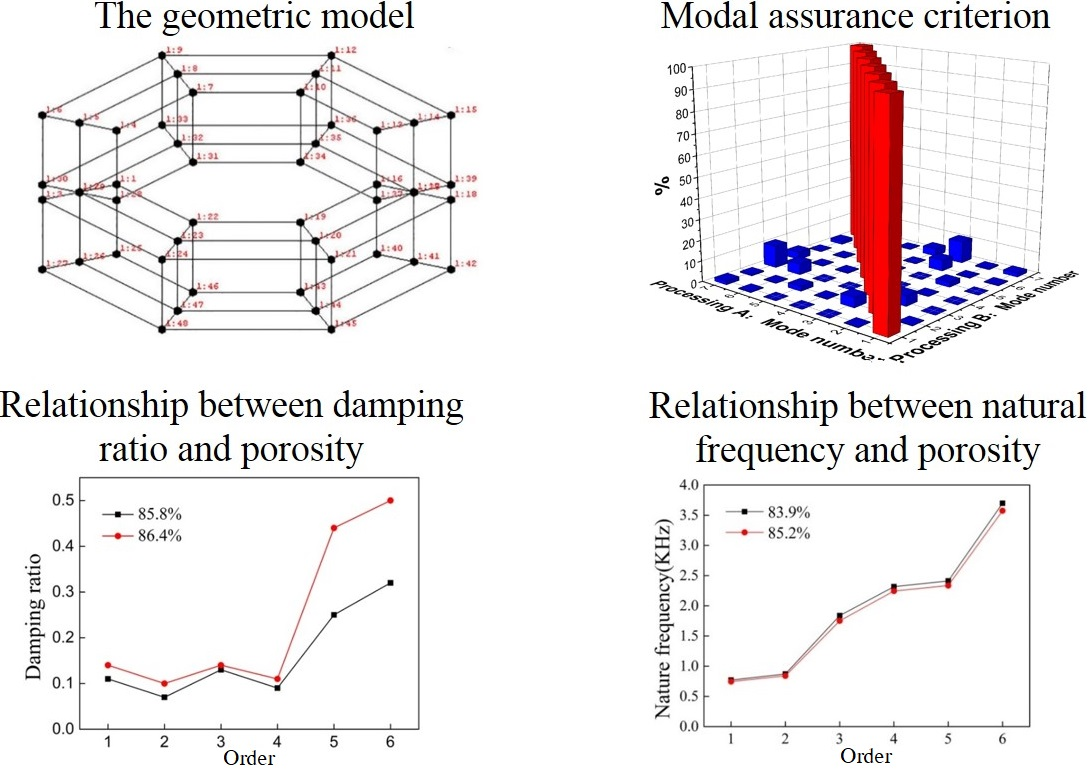#### Highlights

• Modal analysis method can effectively analyze the dynamic characteristics of closed-cell aluminum foam.
• The natural frequency of closed-cell aluminum foam is negatively correlated to porosity and it increases with the increase of sample height when the axial dimension smaller than the radial dimension.
• FEM can be used to study the dynamic characteristic of closed-cell aluminum foam and compared with experiment it has some advantages.

## 1. Introduction

Closed-cell aluminum foam is a new kind of multifunctional metal porous material, which has the characteristics of both metal and bubbles. It’s becoming one of the hot research topics in the current metal material field for its excellent mechanical and physical properties , such as low density, high specific strength, high specific stiffness, high energy absorption, high damping vibration, phonics, electromagnetic shielding and its multi-function compatible . One of its main uses is as damping material; it can effectively reduce the vibration and noise that has a great significance to improve the accuracy and life of equipment as well as the working environment.

Now, some aspects of closed-cell aluminum foam have been deeply researched by experiment and FEM, such as the production process , dynamic and static compression  and energy absorption . For its dynamic characteristics, especially the vibration damping performance mainly concentrated in the damping test referenced the standard of ASTM E756-05. Han et al.  and Liu et al.  have researched the factors influencing the damping property of closed-cell aluminum foam and damping mechanism. Golovin and Sinning  have researched its mechanical damping in a wide range of deformation amplitude. L. Dahil et al.  have studied the relationship between density and damping ratio of foamed aluminum using modal analysis method.

In this article, first, describe the analysis of feature parameters, adopted a new and simple method to analyze the porosity, characteristics of pore. Then using the modal analysis method to research the relationship between dynamic characteristic and feature parameters. Modal assurance criterion (MAC) and coherence function were used to verify the correctness of the model. We also compared the results by Finite element method (FEM). Last, the conclusions.

## 2. Experimental procedures

### 2.1. Feature parameters

The samples were made by melt foaming method and incised by wire-electrode cutting method. The sample size, outside diameter is Ø440 mm, inside diameter is Ø280 mm and the height is unequal.

Porosity is an important parameter to describe the closed-cell aluminum foam. The measurement method of porosity is divided into weighing method and microscopic method. For its simple and high precision, weighing method is commonly used. According to the dimension and weight of the sample, using the Eq. (1) to calculate the porosity:

1

In which, $m$ and $V$ are the mass and volume of the sample, respectively, ${\rho }_{s}$ is the density of matrix material.

The measurement of pore parameters mainly includes direct method and indirect method that using the software to analysis the surface topography of the sample . In this article, we applied a new and simple method to measure the pore parameters. It has the advantages of low cost and high precision. The main equipment is digital camera and the main software is Photoshop, Matlab and Image-Pro Plus. The basic procession is shown in Fig. 1.

Fig. 1The basic procession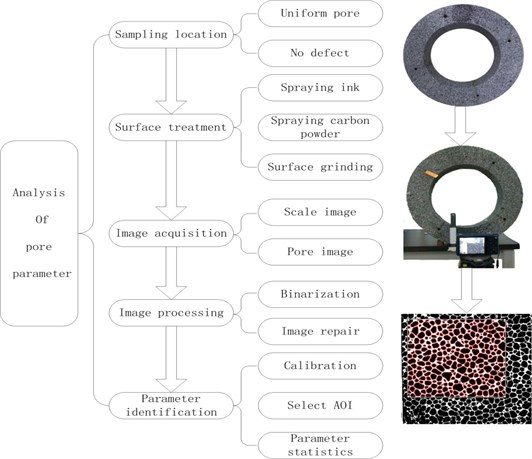### 2.2. Dynamic characteristic test

The dynamic characteristic was performed by experimental modal analysis and FEM. As shown in Fig. 2, the whole testing system composed by four parts; suspension part, shaking part, testing part and software analysis part. The suspension part includes rigid bracket and elastic soft cord to simulate the free boundary condition. The shaking part is impact hammer which is commonly used in the single-input single-output (SISO) modal analysis to produce pulse signal. The testing part is PCB accelerometer and high-speed data acquisition system. The software analysis part is Virtual lab/Modal Analysis software.

Fig. 2The testing system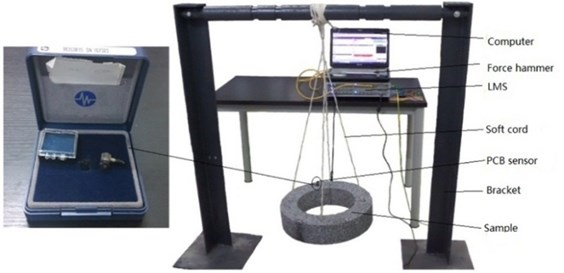In the modeling process, the model is simplified to octagon with 48 nodes and ignores the mounting holes, as shown in Fig. 3. In which point 1:1 to 1:24 is the master node and the rest is the slave node. The PCB acceleration sensor is installed in the 1:3 points. During the experiment, using the force hammer which installed rigid head to beat the other master node, every node beats at least three times. The related experimental parameters as shown in Table 1.

Fig. 3The geometric model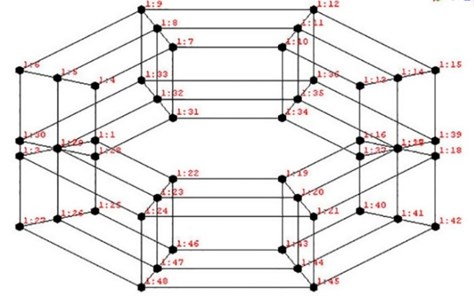Table 1Related experimental parameters

 Force sensor sensitivity PCB sensor sensitivity Bandwidth Resolution Averages Response windowing 4 PC/N 101.7 mv/g 4096 Hz 1 Hz 3 Exponential

MAC was used to test the linear independence of each order. MAC is a good tool to evaluate the modal vector space angle. It can be expressed as follows:

2
$MAC=ma{c}_{ij}=\frac{\left[{\phi }_{i}^{T}{\phi }_{j}\right]}{\left({\phi }_{i}^{T}{\phi }_{i}\right)\left({\phi }_{j}^{T}{\phi }_{j}\right)},$

where and ${\phi }_{j}$ are the corresponding freedom of the $i$ and $j$ order calculation mode, respectively. The smaller of the off-diagonal matrix is the better of the independence of each calculation mode.

In this article, we also used the ABAQUS software to give a modal analysis in order to better verify the testing results. The basic steps of modal analysis in ABAQUS include modeling, select the analysis type, set the corresponding parameters, applying the boundary conditions, solve and results post processing. The parameters used in the simulation are shown in Table 2.

Table 2Parameters used in FEM

 Height Porosity Density (kg/m3) Young’s modulus × 106(Pa) Poisson’s ratio 50 85.8 % 380 428.61 0.28 50 86.4 % 370 401.14 0.28 80 81.9 % 490 603.07 0.28 80 85.0 % 400 484 0.28 120 83.9 % 430 524.36 0.28 120 85.2 % 400 454.67 0.28 150 84.6 % 420 502.18 0.28 150 86.0 % 380 451.43 0.28

## 3. Results and discussion

### 3.1. Feature parameters analysis

Table 3 shows the feature parameters of aluminum foam. It can be found that the porosity is among 81.9 % to 86.42 %, the relative density is among 0.136 to 0.181, the average diameter is among 2.42 to 3.23 mm.

Table 3Parameters of aluminum foam

 No. Height / mm Mass / g Porosity Diameter / mm Relative density 1 50 1761 85.79 % 3.23 0.142 2 50 1665 86.42 % 2.65 0.136 3 80 2930 85.01 % 2.77 0.150 4 80 3540 81.90 % 2.42 0.181 5 120 4694 83.92 % 2.44 0.161 6 120 4334 85.21 % 2.72 0.148 7 150 5629 84.61 % 2.70 0.154 8 150 5132 85.95 % 3.09 0.140

### 3.2.1. Results and validation

In this article, we analyzed the first six orders. As shown in Fig. 4 is the MAC of each sample. Through the MAC it can be found that except the diagonal correlation is 100 %, the other off-diagonal correlation is fewer than 10 % and mostly fewer than 5 %. According the above criteria of MAC, it can be found that the node configuration is reasonable, and each order modal has higher orthogonality.

Fig. 4Modal assurance criterion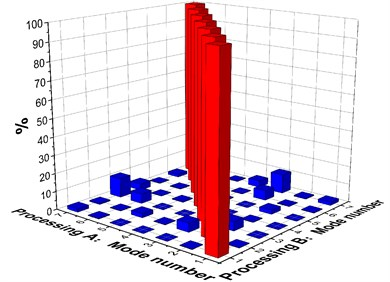a) Sample one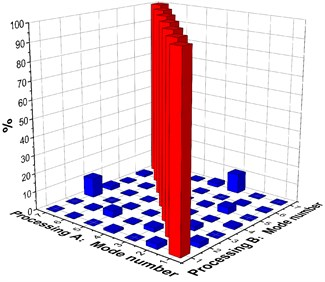b) Sample two

### 3.2.2. Discussion of experimental modal results

(1) Relationship between damping ratio and porosity.

As shown in Fig. 5 is the result of damping ratio of each sample. It can be seen from Fig. 5 each order damping ratio increases with the increase of porosity, the damping ratio and porosity is positively correlated. But in some orders the damping ratio decreases with the increase of porosity for the manufacturing defect.

Fig. 5Relationship between damping ratio and porosity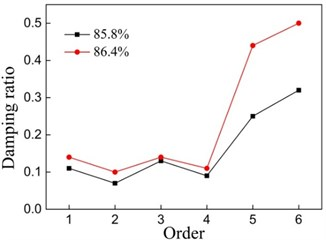a) Sample one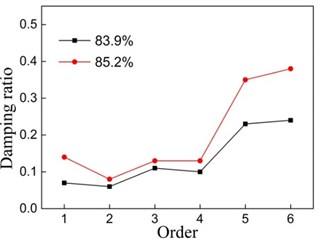b) Sample two

(2) Relationship between natural frequency and porosity.

Material’s vibration damping performance and damping ratio are closely related, but there is another important factor that affects the vibration damping performance is the natural frequency of the sample. As shown in Fig. 6, it can be found that the natural frequency of each order decreases with the increase of porosity and increases with the increase of height of the sample. In this experiment, the sample’s axial dimension is less than the radial dimension, the stiffness of axial increases greater than the increase of mass, its natural frequency is increased with the increase of height.

Fig. 6Relationship between natural frequency and porosity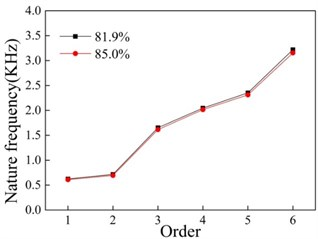a) Sample one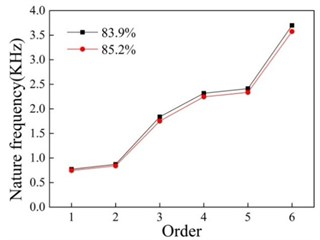b) Sample two

### 3.3. Comparison between experiment and FEM

By comparing the simulation results and experimental results can make a mutual authentication between the two methods. The results are shown in the Table 4. As it can be seen the simulation results can be good fit with the testing results. So, in the next study, we can use the FEM to study its dynamic characteristic.

## 4. Conclusions

First, modal analysis method can effectively analyze the dynamic characteristics of closed-cell aluminum foam. Second, the natural frequency of closed-cell aluminum foam is negatively correlated to porosity and it increases with the increase of sample height when the axial dimension smaller than the radial dimension.

Third, FEM can be used to study the dynamic characteristic of closed-cell aluminum foam and compared with experiment it has some advantages; it can make a further optimization analysis of the sample shape by FEM.

Table 4Comparison between FEM and experiment

 Height Porosity Item First orderfrequency Second orderfrequency Third orderfrequency Forth orderfrequency Fifth orderfrequency Sixth orderfrequency 50 0.8579 Test value 412.521 467.224 1156.314 1569.274 1991.156 2275.407 FEM value 441.43 464.18 1172 1558.6 2041 2259.5 Error 0.07 –0.0065 0.014 –0.0068 0.025 –0.0069 50 0.8642 Test value 403.873 465.858 1147.42 1550.125 1924.597 2261.659 FEM value 432.93 445.16 1144.3 1572.7 1957.4 2166.9 Error 0.072 –0.044 –0.0027 0.015 0.017 –0.042 80 0.819 Test value 624.184 715.786 1652.535 2046.163 2352.014 3219.411 FEM value 627.88 712.81 1855.4 2084.4 2312.3 3259.3 Error 0.0059 –0.0042 0.123 0.019 –0.0017 0.012 80 0.8501 Test value 611.275 694.411 1614.625 2016.47 2312.069 3153.054 FEM value 635.52 649.99 1607.7 2109.8 2314.9 3123.4 Error 0.04 –0.064 –0.0043 0.046 0.0012 –0.0094 120 0.8392 Test value 771.856 871.648 1838.794 2316.829 2412.699 3698.126 FEM value 784.96 869.53 1871 2407.3 2423.7 3701.1 Error 0.017 –0.0024 0.018 0.039 0.0046 0.001 120 0.8521 Test value 745.5 839.099 1751.82 2244.576 2335.451 3574.857 FEM value 767.51 849.5 1806.4 2324.2 2340 3598.4 Error 0.03 0.013 0.03 0.035 0.002 0.007 150 0.8461 Test value 805.083 918.122 1790.455 2343.469 2972.636 3238.733 FEM value 789.37 914.77 1770 2298.6 3034.9 3203.2 Error –0.02 –0.004 –0.01 0.019 0.021 –0.011 150 0.8595 Test value 794.044 895.565 1766.027 2315.208 2931.719 3118.055 FEM value 786.83 911.79 1764.3 2291.2 3025.1 3137.9 Error –0.01 0.018 –0.001 –0.01 0.032 0.007

#### Cited by

Numerical and statistical investigation of the performance of closed-cell aluminium foam as a seismic isolation layer for tunnel linings